# sklearn.preprocessing.StandardScaler¶

class sklearn.preprocessing.StandardScaler(*, copy=True, with_mean=True, with_std=True)[source]

Standardize features by removing the mean and scaling to unit variance.

The standard score of a sample x is calculated as:

z = (x - u) / s

where u is the mean of the training samples or zero if with_mean=False, and s is the standard deviation of the training samples or one if with_std=False.

Centering and scaling happen independently on each feature by computing the relevant statistics on the samples in the training set. Mean and standard deviation are then stored to be used on later data using transform.

Standardization of a dataset is a common requirement for many machine learning estimators: they might behave badly if the individual features do not more or less look like standard normally distributed data (e.g. Gaussian with 0 mean and unit variance).

For instance many elements used in the objective function of a learning algorithm (such as the RBF kernel of Support Vector Machines or the L1 and L2 regularizers of linear models) assume that all features are centered around 0 and have variance in the same order. If a feature has a variance that is orders of magnitude larger than others, it might dominate the objective function and make the estimator unable to learn from other features correctly as expected.

This scaler can also be applied to sparse CSR or CSC matrices by passing with_mean=False to avoid breaking the sparsity structure of the data.

Read more in the User Guide.

Parameters:
copybool, default=True

If False, try to avoid a copy and do inplace scaling instead. This is not guaranteed to always work inplace; e.g. if the data is not a NumPy array or scipy.sparse CSR matrix, a copy may still be returned.

with_meanbool, default=True

If True, center the data before scaling. This does not work (and will raise an exception) when attempted on sparse matrices, because centering them entails building a dense matrix which in common use cases is likely to be too large to fit in memory.

with_stdbool, default=True

If True, scale the data to unit variance (or equivalently, unit standard deviation).

Attributes:
scale_ndarray of shape (n_features,) or None

Per feature relative scaling of the data to achieve zero mean and unit variance. Generally this is calculated using np.sqrt(var_). If a variance is zero, we can’t achieve unit variance, and the data is left as-is, giving a scaling factor of 1. scale_ is equal to None when with_std=False.

New in version 0.17: scale_

mean_ndarray of shape (n_features,) or None

The mean value for each feature in the training set. Equal to None when with_mean=False.

var_ndarray of shape (n_features,) or None

The variance for each feature in the training set. Used to compute scale_. Equal to None when with_std=False.

n_features_in_int

Number of features seen during fit.

New in version 0.24.

feature_names_in_ndarray of shape (n_features_in_,)

Names of features seen during fit. Defined only when X has feature names that are all strings.

New in version 1.0.

n_samples_seen_int or ndarray of shape (n_features,)

The number of samples processed by the estimator for each feature. If there are no missing samples, the n_samples_seen will be an integer, otherwise it will be an array of dtype int. If sample_weights are used it will be a float (if no missing data) or an array of dtype float that sums the weights seen so far. Will be reset on new calls to fit, but increments across partial_fit calls.

scale

Equivalent function without the estimator API.

PCA

Further removes the linear correlation across features with ‘whiten=True’.

Notes

NaNs are treated as missing values: disregarded in fit, and maintained in transform.

We use a biased estimator for the standard deviation, equivalent to numpy.std(x, ddof=0). Note that the choice of ddof is unlikely to affect model performance.

For a comparison of the different scalers, transformers, and normalizers, see examples/preprocessing/plot_all_scaling.py.

Examples

>>> from sklearn.preprocessing import StandardScaler
>>> data = [[0, 0], [0, 0], [1, 1], [1, 1]]
>>> scaler = StandardScaler()
>>> print(scaler.fit(data))
StandardScaler()
>>> print(scaler.mean_)
[0.5 0.5]
>>> print(scaler.transform(data))
[[-1. -1.]
[-1. -1.]
[ 1.  1.]
[ 1.  1.]]
>>> print(scaler.transform([[2, 2]]))
[[3. 3.]]


Methods

 fit(X[, y, sample_weight]) Compute the mean and std to be used for later scaling. fit_transform(X[, y]) Fit to data, then transform it. get_feature_names_out([input_features]) Get output feature names for transformation. get_params([deep]) Get parameters for this estimator. inverse_transform(X[, copy]) Scale back the data to the original representation. partial_fit(X[, y, sample_weight]) Online computation of mean and std on X for later scaling. set_output(*[, transform]) Set output container. set_params(**params) Set the parameters of this estimator. transform(X[, copy]) Perform standardization by centering and scaling.
fit(X, y=None, sample_weight=None)[source]

Compute the mean and std to be used for later scaling.

Parameters:
X{array-like, sparse matrix} of shape (n_samples, n_features)

The data used to compute the mean and standard deviation used for later scaling along the features axis.

yNone

Ignored.

sample_weightarray-like of shape (n_samples,), default=None

Individual weights for each sample.

New in version 0.24: parameter sample_weight support to StandardScaler.

Returns:
selfobject

Fitted scaler.

fit_transform(X, y=None, **fit_params)[source]

Fit to data, then transform it.

Fits transformer to X and y with optional parameters fit_params and returns a transformed version of X.

Parameters:
Xarray-like of shape (n_samples, n_features)

Input samples.

yarray-like of shape (n_samples,) or (n_samples, n_outputs), default=None

Target values (None for unsupervised transformations).

**fit_paramsdict

Returns:
X_newndarray array of shape (n_samples, n_features_new)

Transformed array.

get_feature_names_out(input_features=None)[source]

Get output feature names for transformation.

Parameters:
input_featuresarray-like of str or None, default=None

Input features.

• If input_features is None, then feature_names_in_ is used as feature names in. If feature_names_in_ is not defined, then the following input feature names are generated: ["x0", "x1", ..., "x(n_features_in_ - 1)"].

• If input_features is an array-like, then input_features must match feature_names_in_ if feature_names_in_ is defined.

Returns:
feature_names_outndarray of str objects

Same as input features.

get_params(deep=True)[source]

Get parameters for this estimator.

Parameters:
deepbool, default=True

If True, will return the parameters for this estimator and contained subobjects that are estimators.

Returns:
paramsdict

Parameter names mapped to their values.

inverse_transform(X, copy=None)[source]

Scale back the data to the original representation.

Parameters:
X{array-like, sparse matrix} of shape (n_samples, n_features)

The data used to scale along the features axis.

copybool, default=None

Copy the input X or not.

Returns:
X_tr{ndarray, sparse matrix} of shape (n_samples, n_features)

Transformed array.

partial_fit(X, y=None, sample_weight=None)[source]

Online computation of mean and std on X for later scaling.

All of X is processed as a single batch. This is intended for cases when fit is not feasible due to very large number of n_samples or because X is read from a continuous stream.

The algorithm for incremental mean and std is given in Equation 1.5a,b in Chan, Tony F., Gene H. Golub, and Randall J. LeVeque. “Algorithms for computing the sample variance: Analysis and recommendations.” The American Statistician 37.3 (1983): 242-247:

Parameters:
X{array-like, sparse matrix} of shape (n_samples, n_features)

The data used to compute the mean and standard deviation used for later scaling along the features axis.

yNone

Ignored.

sample_weightarray-like of shape (n_samples,), default=None

Individual weights for each sample.

New in version 0.24: parameter sample_weight support to StandardScaler.

Returns:
selfobject

Fitted scaler.

set_output(*, transform=None)[source]

Set output container.

See Introducing the set_output API for an example on how to use the API.

Parameters:
transform{“default”, “pandas”}, default=None

Configure output of transform and fit_transform.

• "default": Default output format of a transformer

• "pandas": DataFrame output

• None: Transform configuration is unchanged

Returns:
selfestimator instance

Estimator instance.

set_params(**params)[source]

Set the parameters of this estimator.

The method works on simple estimators as well as on nested objects (such as Pipeline). The latter have parameters of the form <component>__<parameter> so that it’s possible to update each component of a nested object.

Parameters:
**paramsdict

Estimator parameters.

Returns:
selfestimator instance

Estimator instance.

transform(X, copy=None)[source]

Perform standardization by centering and scaling.

Parameters:
X{array-like, sparse matrix of shape (n_samples, n_features)

The data used to scale along the features axis.

copybool, default=None

Copy the input X or not.

Returns:
X_tr{ndarray, sparse matrix} of shape (n_samples, n_features)

Transformed array.

## Examples using sklearn.preprocessing.StandardScaler¶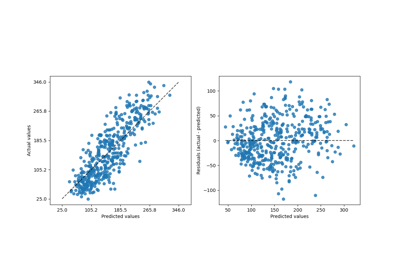Release Highlights for scikit-learn 1.2

Release Highlights for scikit-learn 1.2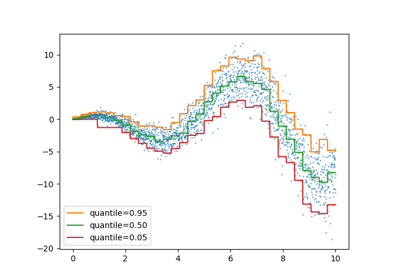Release Highlights for scikit-learn 1.1

Release Highlights for scikit-learn 1.1Release Highlights for scikit-learn 1.0

Release Highlights for scikit-learn 1.0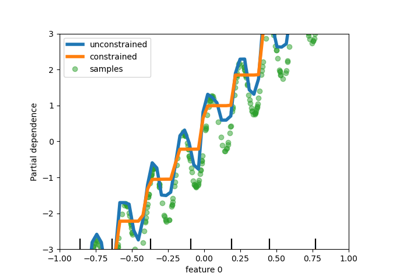Release Highlights for scikit-learn 0.23

Release Highlights for scikit-learn 0.23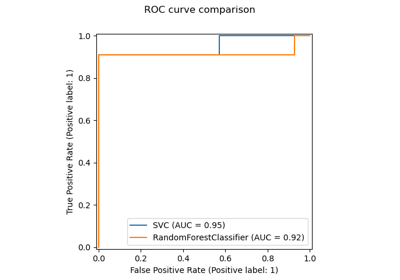Release Highlights for scikit-learn 0.22

Release Highlights for scikit-learn 0.22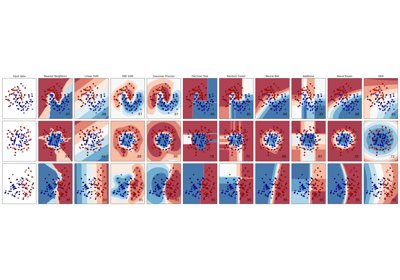Classifier comparison

Classifier comparison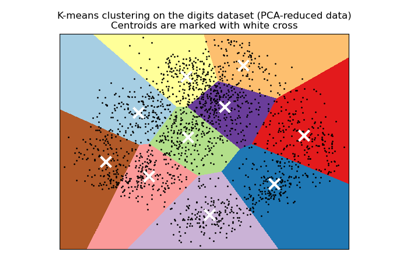A demo of K-Means clustering on the handwritten digits data

A demo of K-Means clustering on the handwritten digits data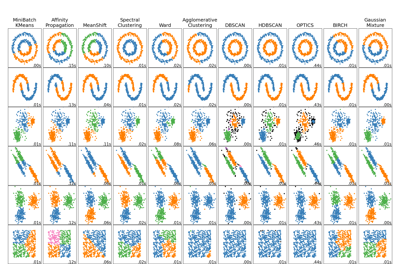Comparing different clustering algorithms on toy datasets

Comparing different clustering algorithms on toy datasets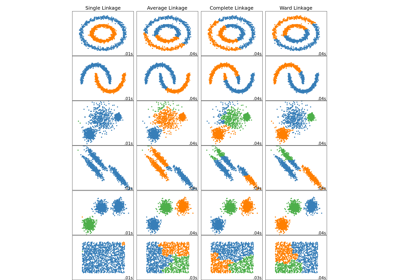Comparing different hierarchical linkage methods on toy datasets

Comparing different hierarchical linkage methods on toy datasets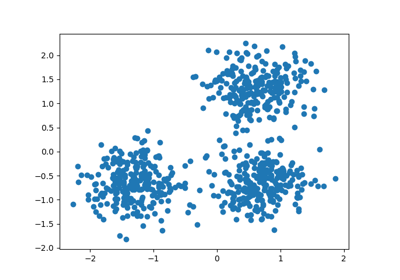Demo of DBSCAN clustering algorithm

Demo of DBSCAN clustering algorithm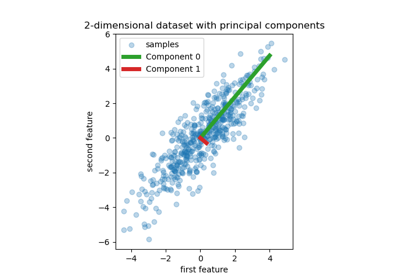Principal Component Regression vs Partial Least Squares Regression

Principal Component Regression vs Partial Least Squares Regression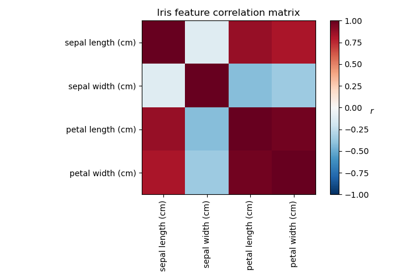Factor Analysis (with rotation) to visualize patterns

Factor Analysis (with rotation) to visualize patterns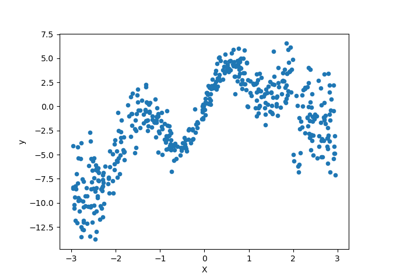Combine predictors using stacking

Combine predictors using stacking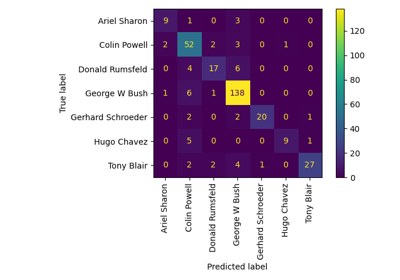Faces recognition example using eigenfaces and SVMs

Faces recognition example using eigenfaces and SVMs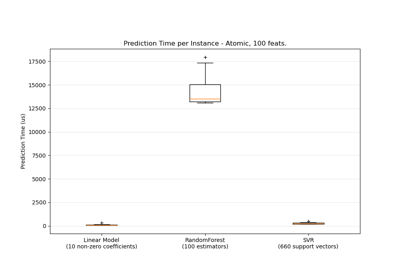Prediction Latency

Prediction Latency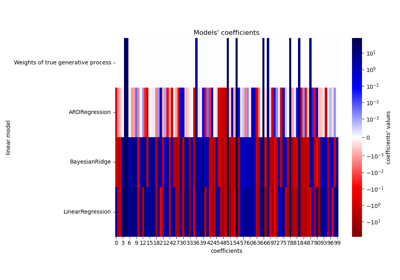Comparing Linear Bayesian Regressors

Comparing Linear Bayesian Regressors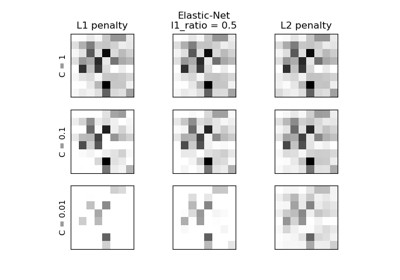L1 Penalty and Sparsity in Logistic Regression

L1 Penalty and Sparsity in Logistic Regression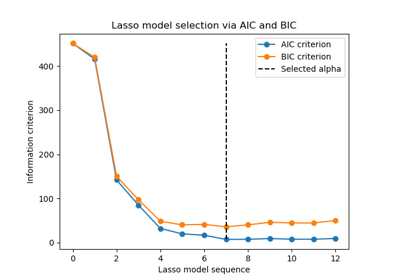Lasso model selection via information criteria

Lasso model selection via information criteria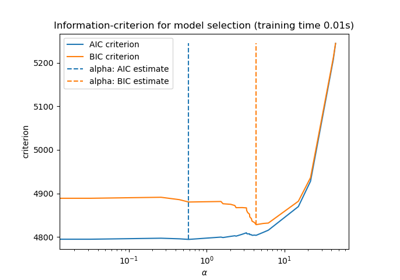Lasso model selection: AIC-BIC / cross-validation

Lasso model selection: AIC-BIC / cross-validationMNIST classification using multinomial logistic + L1

MNIST classification using multinomial logistic + L1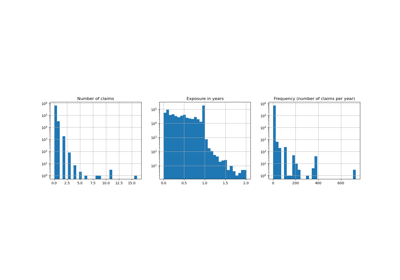Poisson regression and non-normal loss

Poisson regression and non-normal loss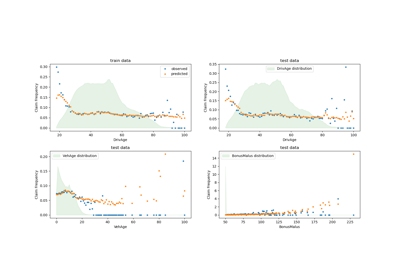Tweedie regression on insurance claims

Tweedie regression on insurance claims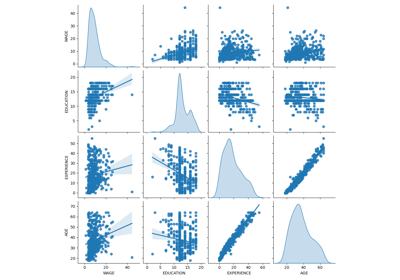Common pitfalls in the interpretation of coefficients of linear models

Common pitfalls in the interpretation of coefficients of linear models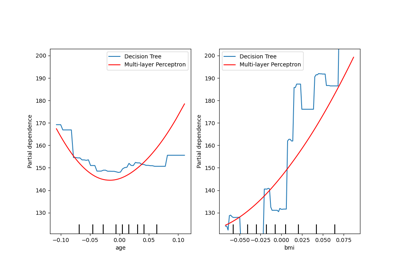Displaying Pipelines

Displaying PipelinesDisplaying estimators and complex pipelines

Displaying estimators and complex pipelines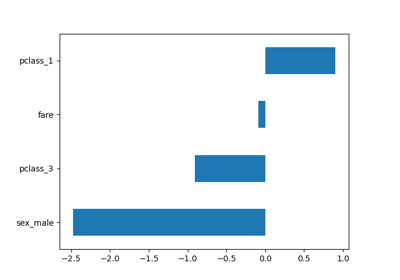Introducing the set_output API

Introducing the set_output API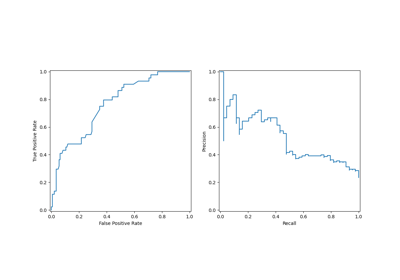Visualizations with Display Objects

Visualizations with Display Objects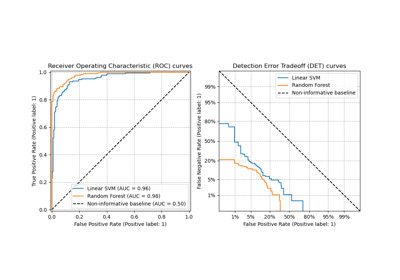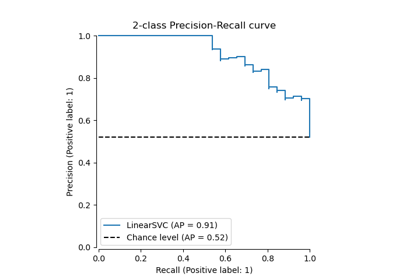Precision-Recall

Precision-Recall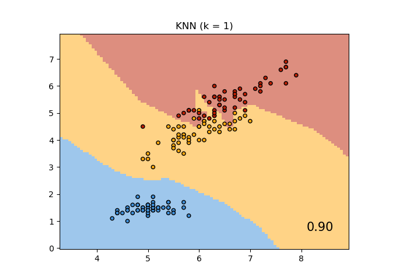Comparing Nearest Neighbors with and without Neighborhood Components Analysis

Comparing Nearest Neighbors with and without Neighborhood Components Analysis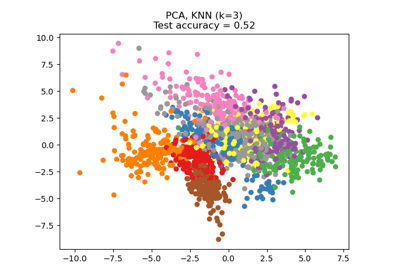Dimensionality Reduction with Neighborhood Components Analysis

Dimensionality Reduction with Neighborhood Components Analysis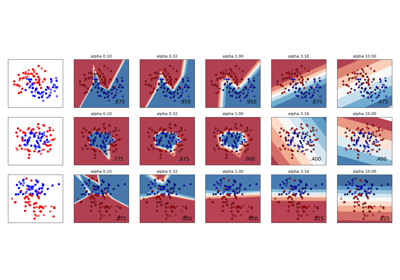Varying regularization in Multi-layer Perceptron

Varying regularization in Multi-layer PerceptronColumn Transformer with Mixed Types

Column Transformer with Mixed Types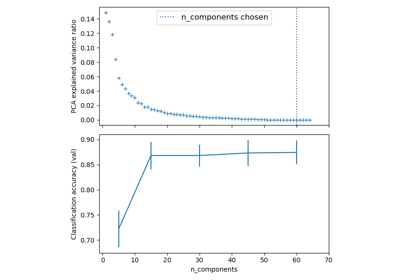Pipelining: chaining a PCA and a logistic regression

Pipelining: chaining a PCA and a logistic regression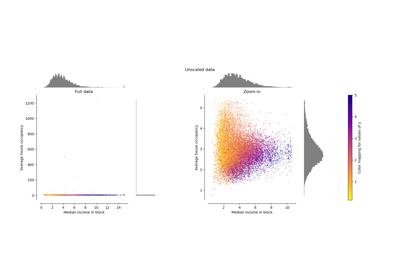Compare the effect of different scalers on data with outliers

Compare the effect of different scalers on data with outliers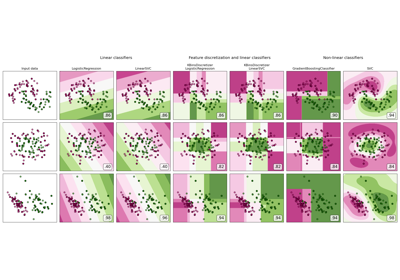Feature discretization

Feature discretization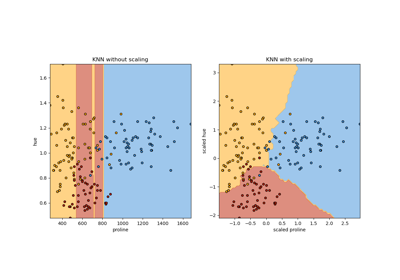Importance of Feature Scaling

Importance of Feature Scaling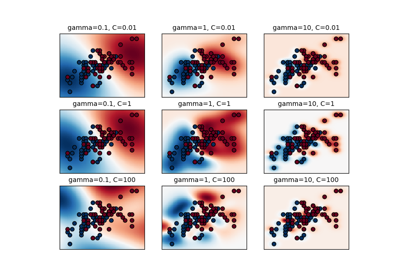RBF SVM parameters

RBF SVM parameters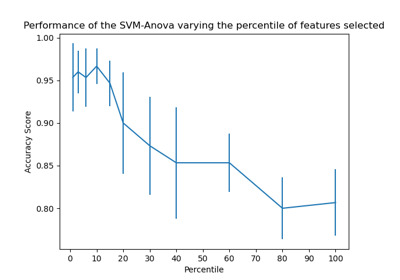SVM-Anova: SVM with univariate feature selection

SVM-Anova: SVM with univariate feature selection